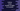# Count number of digits in a number in Python## Count the number of digits in a number using python :

Using python, count the number of digits in a number. In this tutorial, we will learn how to count the total number of digits in a number using python. The program will get the input from the user and print out the result.

We will show you two different ways to calculate total digits in a number.

## Solution 1 (using a while loop) :

The idea behind this solution is to keep deleting the rightmost digit of the number one by one until the number becomes zero.

Following algorithm we are going to use for this approach :

1. Take the user input and store it in a variable.
2. Create one counter variable to hold the total number count. Initialize this variable to zero at the start of the program.
3. Using a while loop, delete the rightmost digit of the number or convert the number to this new number. For example, if the number is 123, convert it to 12, then convert it to 1 and finally 0.
4. Increment the counter variable by 1 on each conversion. Do it until the number becomes zero. At the end of the while loop, this counter variable will hold the total digit count of the number.
5. Print the counter variable.

### Python program :

``````#example 1

count = 0
number = int(input("Enter a number "))

while (number > 0):
number = number//10
count = count + 1

print ("Total number of digits : ",count)

#example 2
count = 0
number = int(input("Enter a number "))

print ("Total number of digits : ",len(str(abs(number))))``````

You can also download this program from here.

### Explanation :

1. The code is similar to the algorithm we have explained above. ‘count’ is the counter variable used to hold the total number of digits in the number. This variable is initialized to zero at the beginning.
2. We are using the input() method to read the user input. This method returns a string, we are wrapping this value with int() to get the integer value of the number user has entered. This value is stored in the ‘number’ variable.
3. The ‘while loop’ will run until the value of ‘number’ is greater than zero.
4. Inside the loop, we are dividing the number by 10 and assigning this new value to the number. If the value is 123, it will be 12 after the division. It is same as like deleting the last digit of the number.
5. Increment the value of count by 1 after the conversion. Eventually, the ‘number’ variable will become zero and the loop will stop.
6. Print the value of the ’count’ at the end of the program.

## Solution 2 ( Using inbuilt methods ) :

Instead of using a while loop, we can directly convert the integer to a string and get the length of the string. In python, getting the length of a string is easy by using the ’len()’ method. For converting a number to a string, we can use the ’str()’ method.

‘abs’ is used to get the absolute value of a number. i.e. to handle the case even if the number is negative.

For example, if our input value is ’-123‘, abs() will convert it to ’123‘. str() will convert it to a string and len() will return the length of the string or 3.

### Python program :

``````#example 1

count = 0
number = int(input("Enter a number "))

while (number > 0):
number = number//10
count = count + 1

print("Total number of digits : ", count)

#example 2
count = 0
number = int(input("Enter a number "))

print("Total number of digits : ", len(str(abs(number))))``````

You can also download this program from here.